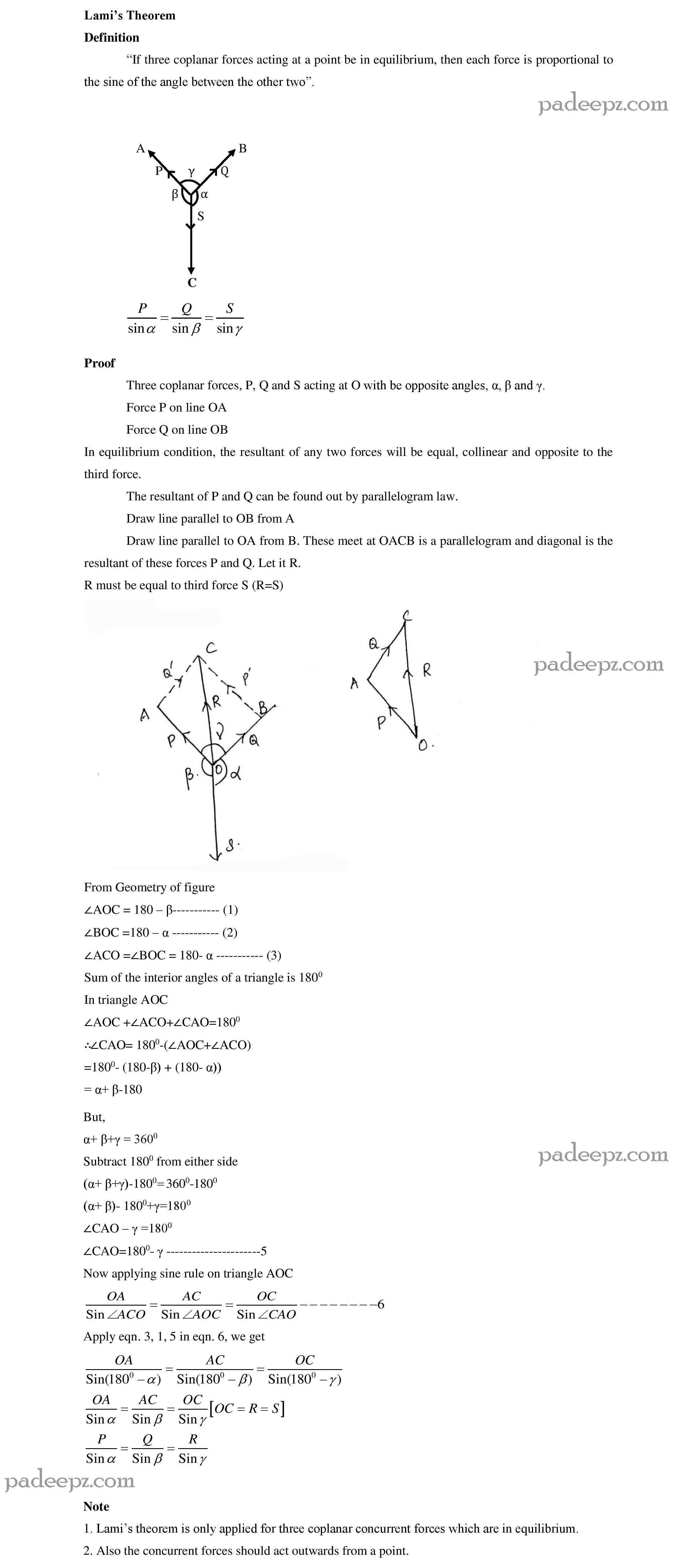Categories

# GE8292 Question Bank Engineering mechanics

GE8292 Question Bank Engineering mechanics EM for regulation 2017 Anna University pdf free download. Engineering mechanics Question Bank EM Anna University pdf free download.

## Sample GE8292 Question Bank Engineering mechanics EM:

1. Resolve the 100N force acting 30° to horizontal into two
components, one along horizontal and other along 120° to
horizontal GE8292 Question Bank Engineering mechanics EM

2. State the Second Law of Newton. BT-1 Remembering

3. Write the equations of equilibrium of a coplanar system of forces. BT-1

4. State Lami’s theorem with a neat sketch? BT-1 Remembering GE8292 Question Bank Engineering mechanics EM

5. State the Parallelogram law of forces? BT-1 Remembering

6. State the triangular law of forces? BT-1 Remembering

7. Define principle of transmissibility. BT-1 Remembering

8. Distinguish the following system of forces with a suitable sketch.

a) Coplanar b) Collinear.
BT-2 Understanding GE8292 Question Bank Engineering mechanics EM

9. Find the resultant of Concurrent forces F̄
1 = 2i+3j-4k ,

2 = 5i-4j+6k & F̄
3 = -2i+3j-2k.
BT-2 Understanding

10. What differences exist between Kinetics and Kinematics. BT-2 Understanding

11. State the Gravitational Law of Newton. BT-2 Understanding

12. Solve the following: A force vector F= 700i + 1500j is applied to a
bolt. Determine the magnitude of the force and angle it forms with
the horizontal.
BT-3 Applying GE8292 Question Bank Engineering mechanics EM

13. Solve the following: A force of magnitude 50 KN is acting along
the line joining A (2,0,6) and B (3,-2,0)m.
Write the vector form of the force.
BT-3 Applying

14. Solve the following: Two forces of magnitude 50 KN and 80 KN
are acting on a particle, such that the angle between the two is
135°. If both the force are acting away from the particle, calculate GE8292 Question Bank Engineering mechanics EM

 Subject name ENGINEERING MECHANICS Semester 2 Regulation 2017 regulation Subject Code GE8292